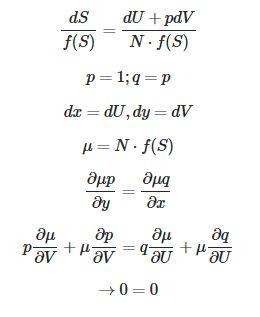# Integrating Factor: Need Help Solving Excersice?

• B4cklfip

#### B4cklfip

Homework Statement
Show that if N is an integrating factor also N*f(S) is an integrating factor.
Relevant Equations
##dS = \frac{dU+pdV}{N}##
I'm not sure if that is the right way to solve this excersice. Can someone maybe help and tell me if this calculation proofs the statement ?Homework Statement:: Show that if N is an integrating factor also N*f(S) is an integrating factor.
Relevant Equations:: ##dS = \frac{dU+pdV}{N}##

I'm not sure if that is the right way to solve this excersice. Can someone maybe help and tell me if this calculation proofs the statement ?

View attachment 263972
First off, ending with 0 = 0 doesn't do you any good.
Second, I'm having a hard time trying to follow what you're doing. Why are you introducing q in the 2nd line and dx and dy in the 3rd line?
What is the differential equation you're trying to solve? Is it Udx + Vdy = 0?
You are given that N is an integrating factor. How have you used it? The basic idea is that U(x, y)dx + V(x, y)dy = 0 is not an exact differential equation, multiplying by an integrating factor causes the new equation to be exact.